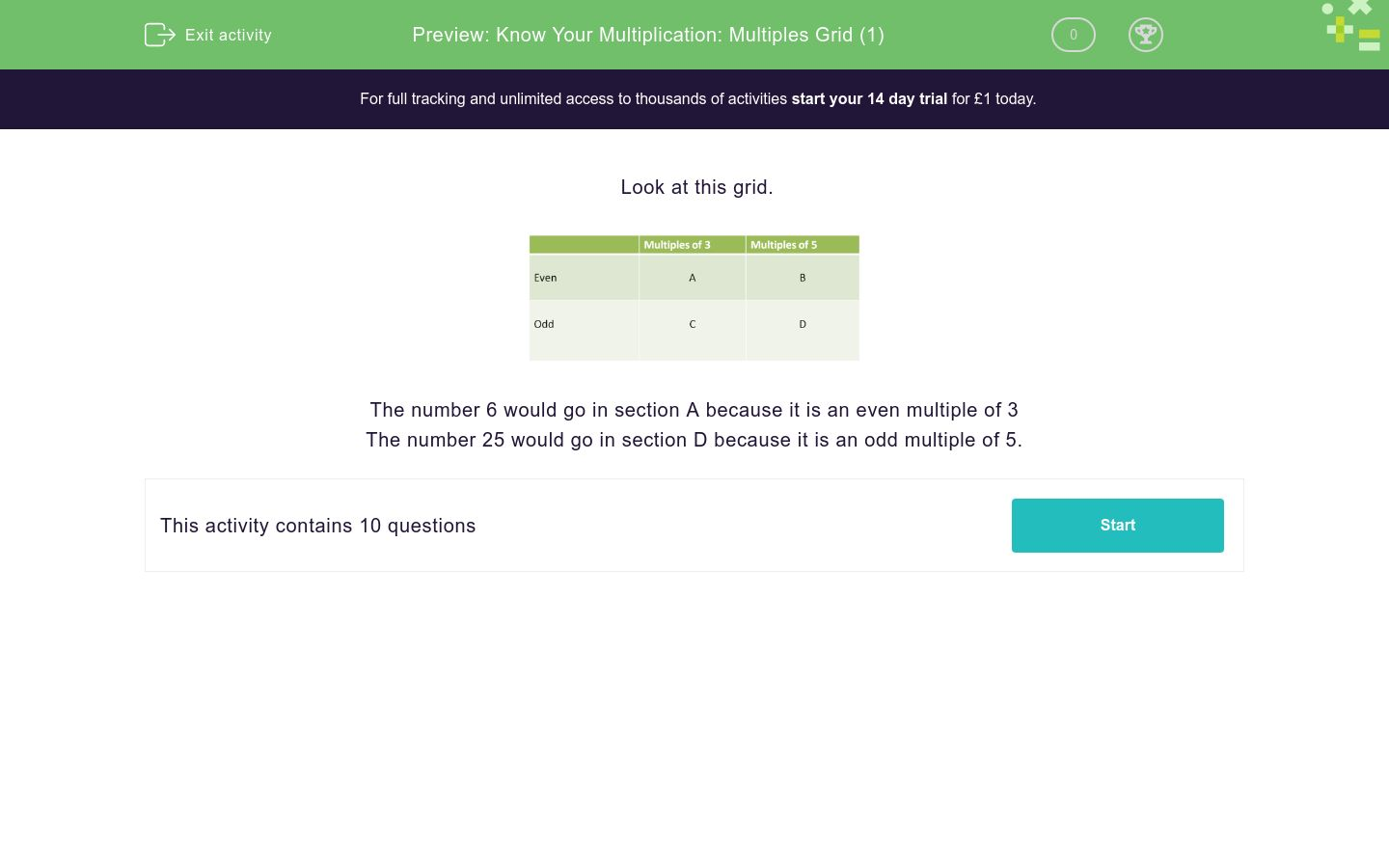Know Your Multiplication: Multiples Grid (1)

In this worksheet, students decide where to place numbers in a grid of odd and even multiples.Key stage:  KS 2

Curriculum topic:  Number: Multiplication and Division

Curriculum subtopic:  Solve Multiplication Problems

Difficulty level:QUESTION 1 of 10

Look at this grid.The number 6 would go in section A because it is an even multiple of 3

The number 25 would go in section D because it is an odd multiple of 5.

In which section should the following number be placed on this grid.

20A

B

C

D

In which section should the following number be placed on this grid.

21A

B

C

D

In which section should the following number be placed on this grid.

18A

B

C

D

In which section should the following number be placed on this grid.

50A

B

C

D

In which section should the following number be placed on this grid.

65A

B

C

D

In which section should the following number be placed on this grid.

63A

B

C

D

In which section should the following number be placed on this grid.

48A

B

C

D

In which section should the following number be placed on this grid.

130A

B

C

D

In which section should the following number be placed on this grid.

125A

B

C

D

In which section should the following number be placed on this grid.

84A

B

C

D

• Question 1

In which section should the following number be placed on this grid.

20B
• Question 2

In which section should the following number be placed on this grid.

21C
• Question 3

In which section should the following number be placed on this grid.

18A
• Question 4

In which section should the following number be placed on this grid.

50B
• Question 5

In which section should the following number be placed on this grid.

65D
• Question 6

In which section should the following number be placed on this grid.

63C
• Question 7

In which section should the following number be placed on this grid.

48A
• Question 8

In which section should the following number be placed on this grid.

130B
• Question 9

In which section should the following number be placed on this grid.

125D
• Question 10

In which section should the following number be placed on this grid.

84